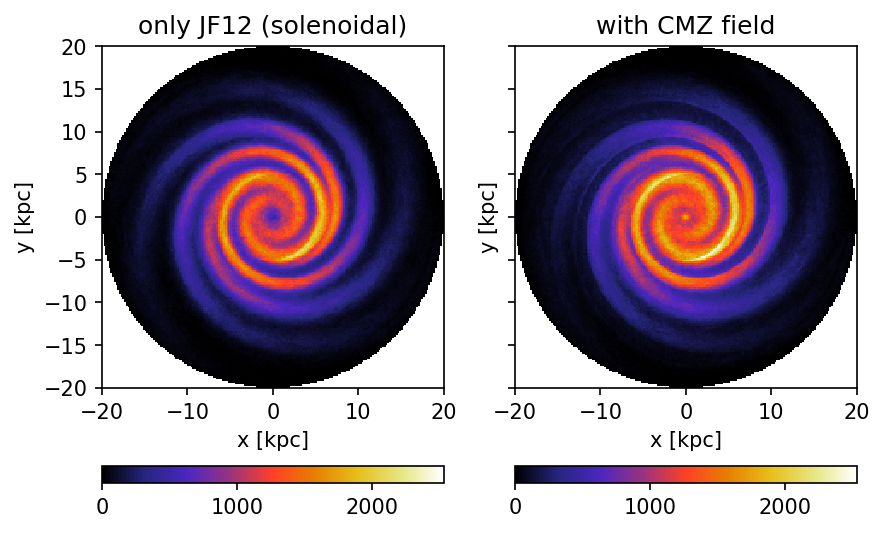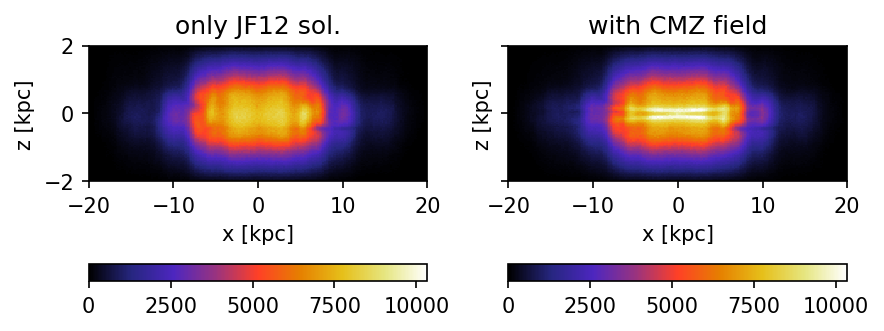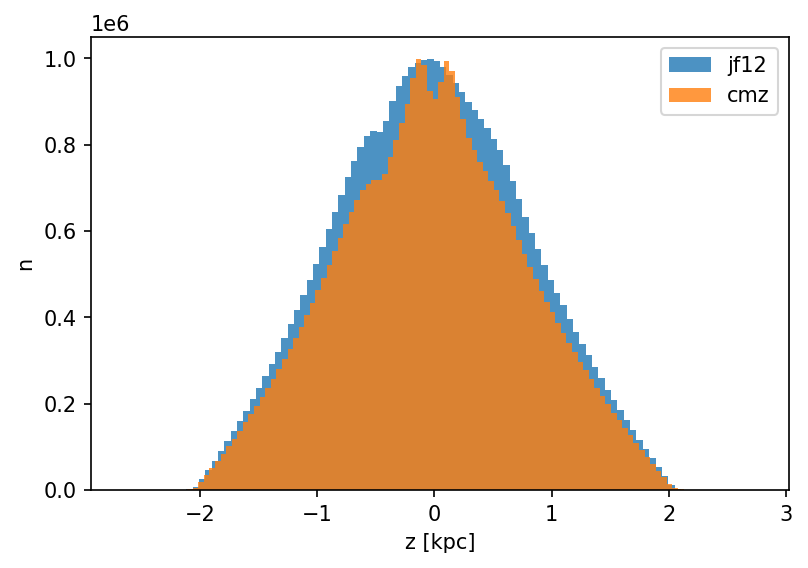# Example for Galactic Propagation

This is a reduced version of the example presented in section 3.1 of the CRPropa 3.2 paper R. Alves Batista et al. JCAP09 (2022) 035.

In this example anisotropic diffuion in different magnetic field configurations is considerd. We use the default values for the parallel diffusion coeficient $$\kappa_\parallel = \kappa_0 \left(\frac{E}{4 \, \mathrm{GeV}}\right)^\alpha$$ with $$\kappa_0 = 6.1\times 10^{28} \mathrm{m^2/s}$$ and $$\alpha = \frac{1}{3}$$ as well for the anisotropy parameter $$\epsilon = \kappa_\perp / \kappa_\parallel = 0.1$$. We test the JF12Solenoidal field by Kleimann et al. ApJ 877 (2019) 76 one time alone and once with the superposition of the inter cloud component of the CMZField by Guenduez et al. A&A 644 (2020) A71.

We simulate Protons with a fixed energy of $$E_p = 10$$ TeV where the source positions follow the SourcePulsarDistribution.

To calculate the stationary solution we follow the weighting approach presented in Merten et al. JCAP 06 (2017) 046. Therefore, we use the ObserverTimeEvolution with $$n = 100$$ steps and $$\Delta t = 5 \, \mathrm{kpc} / c$$.

The simulation volume is limited by a cylinder with the height of $$z = \pm 2 \, \mathrm{kpc}$$ over the Galactic plane and a Galactocentric radius of $$r_\mathrm{gc} = 20 \, \mathrm{kpc}$$.

## import of packages

:

from crpropa import *

import numpy as np
import pandas as pd
import matplotlib.pyplot as plt


## Simulation

This simulation uses a reduced number of candidates compared to the one presented in the paper. Here, only $$5 \times 10^5$$ pseudo-particles are simulated. The plots for the CRPropa3.2 paper are produced with $$10^8$$ particles and a finer grid for the plots. The number of simulated pseudo-particles can be changed in cell below. With the reduced particle number this example should take about four minutes for each simulation on a 12 core computer.

:

def simulation(field, name):
""" Runs the simulation with different field configuration

field:  magnetic field
name:   simulation name for output naming
"""
sim = ModuleList()
sim.setShowProgress(True)

# Propagation

# Observer and Output
out = TextOutput(name + ".txt")
out.setLengthScale(kpc)
out.disableAll()
out.enable(Output.CurrentPositionColumn)

nStep = 100
deltaStep = 5 * kpc
obs = Observer()
time_evolution = ObserverTimeEvolution(deltaStep, deltaStep, nStep)
obs.setDeactivateOnDetection(False) # propagate candidates after detection
obs.onDetection(out)

# Boundary
sim.add(MaximumTrajectoryLength(nStep * deltaStep)) # limit propagation time, no detection afterwards possible
rMax, zMax = 20 * kpc, 2 * kpc
outer_bound = CylindricalBoundary(Vector3d(0), 2 * zMax, rMax)

# Source
source = Source()

# Number of simulated candidates
Npart = int(5e5)

# run simulation
sim.run(source, Npart)

:

# only JF12Solenoidal
jf12 = JF12FieldSolenoidal()
simulation(jf12, "jf12")

# combined with CMZfield
cmz = CMZField()
cmz.setUseMCField(False)
cmz.setUseICField(True) # only use largescale field
cmz.setUseNTFField(False)
field = MagneticFieldList()
simulation(field, "combined")

crpropa::ModuleList: Number of Threads: 8
Run ModuleList
Started Thu Nov 24 13:41:06 2022 : [ Finished ] 100%    Needed: 00:05:03  - Finished at Thu Nov 24 13:46:09 2022
Run ModuleList
Started Thu Nov 24 13:46:09 2022 : [ Finished ] 100%    Needed: 00:05:39  - Finished at Thu Nov 24 13:51:48 2022



## Analysis

:

# Load data
names = ["X", "Y", "Z"]
data_jf12 = pd.read_csv("jf12.txt", names = names, delimiter = "\t", comment = "#")
data_cmz = pd.read_csv("combined.txt", names = names, delimiter ="\t", comment= "#")


### Face-on view of the Milky Way.

In the paper more bins are used. The reduction of bins here is due to the lower number of propagated candidates.

:

bins = np.linspace(-20, 20, 201) # In the paper 300 bins are used # np.linspace(-20, 20, 301)
binMid = (bins[1:] + bins[:-1])/2

nJF12 = np.histogram2d(data_jf12[abs(data_jf12.Z) < 0.3].X, data_jf12[abs(data_jf12.Z) < 0.3].Y, bins = [bins, bins]).T
nCMZ = np.histogram2d(data_cmz[abs(data_cmz.Z) < 0.3].X, data_cmz[abs(data_cmz.Z) < 0.3].Y, bins = [bins, bins]).T

# restrict to simulaiton volume
vmax = np.max([nJF12.max(), nCMZ.max()])
mXY, mYX = np.meshgrid(binMid, binMid)
boolR = ((mXY**2 + mYX**2) > 20**2)
nJF12[boolR] = np.nan
nCMZ[boolR] = np.nan

fig, (ax1, ax2) = plt.subplots(1,2, sharex=True, sharey=True, dpi = 150)

p1 = ax1.pcolormesh(binMid, binMid, nJF12, cmap = plt.cm.CMRmap, vmax=vmax)
ax1.set_title("only JF12 (solenoidal)")

p2 = ax2.pcolormesh(binMid, binMid, nCMZ, cmap = plt.cm.CMRmap, vmax=vmax)
ax2.set_title("with CMZ field")

for ax in (ax1, ax2):
ax.set_aspect("equal")
ax.set_xlabel("x [kpc]")
ax.set_ylabel("y [kpc]")

plt.colorbar(p1, orientation = "horizontal", ax = ax1)
plt.colorbar(p2, orientation = "horizontal", ax = ax2)

fig.tight_layout()
plt.show()### Edge-on view of the Milky Way

Additional plot of a edge on view of the Milky Way. Here, all data are integrated over the y-axis.

:

binsZ = np.linspace(-2, 2, 101)
binMidZ = (binsZ[1:] + binsZ[:-1])/2

nJF12 = np.histogram2d(data_jf12.X, data_jf12.Z, bins = [bins, binsZ]).T
nCMZ = np.histogram2d(data_cmz.X, data_cmz.Z, bins = [bins, binsZ]).T

vmax = np.max([nJF12.max(), nCMZ.max()])

fig, (ax1, ax2) = plt.subplots(1,2, sharex=True, sharey=True, dpi = 150)

p1 = ax1.pcolormesh(bins, binsZ, nJF12, cmap = plt.cm.CMRmap, vmax=vmax)
ax1.set_title("only JF12 sol.")

p2 = ax2.pcolormesh(bins, binsZ, nCMZ, cmap = plt.cm.CMRmap, vmax=vmax)
ax2.set_title("with CMZ field")

for ax in (ax1, ax2):
ax.set_aspect(4)
ax.set_xlabel("x [kpc]")
ax.set_ylabel("z [kpc]")

plt.colorbar(p1, orientation = "horizontal", ax = ax1)
plt.colorbar(p2, orientation = "horizontal", ax = ax2)

fig.tight_layout()
plt.show()### Distribution along the z-axis

Additional plot showing the distribution over the z-axis.

:

plt.figure(dpi = 150)
plt.hist(data_jf12.Z, bins = 100, alpha = .8, label="jf12")
plt.hist(data_cmz.Z, bins = 100, label="cmz", alpha = .8)
plt.xlabel("z [kpc]")
plt.ylabel("n")
plt.legend()
plt.show()